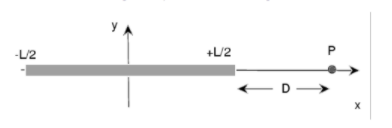# Problem: A charged rod of length L = 5.60 m lies centered on the x axis as shown. The rod has a linear charge density which varies according to λ= ax where a = -58.3 µC/m2.A. What is the total charge on the rod?B. What is the x component of the electric field at a point on the x-axis a distance of D = 7.80 from the end of the rod?

###### FREE Expert Solution

In this problem, we'll consider the element of length, dx.

So, we get the total charge as:

$\overline{){\mathbf{q}}{\mathbf{=}}{\mathbf{\int }}{\mathbf{\lambda }}{\mathbf{x}}{\mathbf{d}}{\mathbf{x}}}$

The electric field due to a point charge:

$\overline{){\mathbf{E}}{\mathbf{=}}\frac{\mathbf{k}\mathbf{q}}{{\mathbf{r}}^{\mathbf{2}}}}$

A.

The linear charge density is given to be:

λ = ax, but a = -58.3 µC/m2 = -58.3 × 10-6 C/m2

Charge on the element of length, dx

dq = λdx = axdx

Total charge on the rod is:

92% (187 ratings)###### Problem Details

A charged rod of length L = 5.60 m lies centered on the x axis as shown. The rod has a linear charge density which varies according to λ= ax where a = -58.3 µC/m2.A. What is the total charge on the rod?

B. What is the x component of the electric field at a point on the x-axis a distance of D = 7.80 from the end of the rod?

Frequently Asked Questions

What scientific concept do you need to know in order to solve this problem?

Our tutors have indicated that to solve this problem you will need to apply the Electric Fields in Conductors concept. You can view video lessons to learn Electric Fields in Conductors. Or if you need more Electric Fields in Conductors practice, you can also practice Electric Fields in Conductors practice problems.